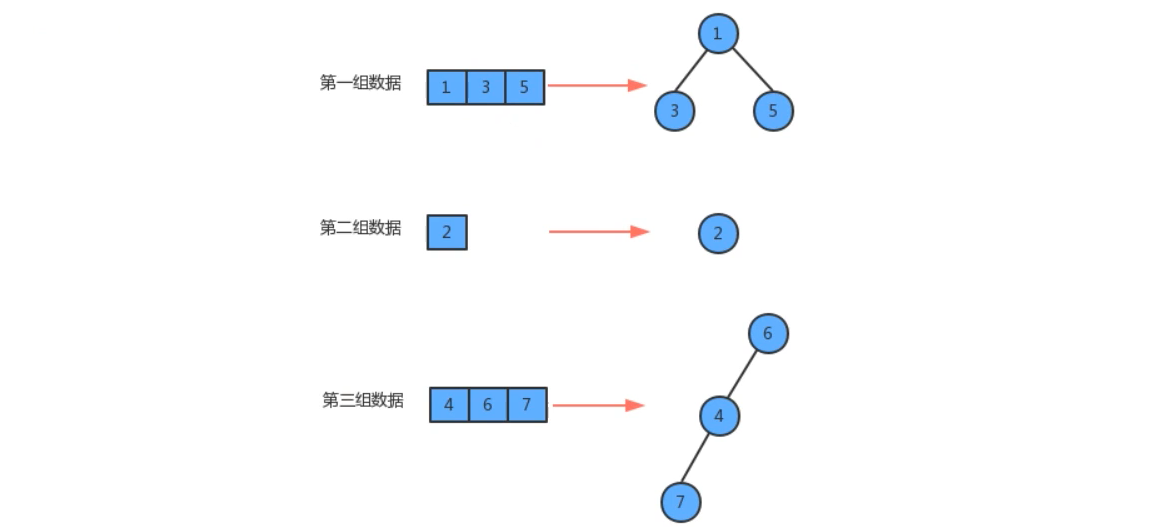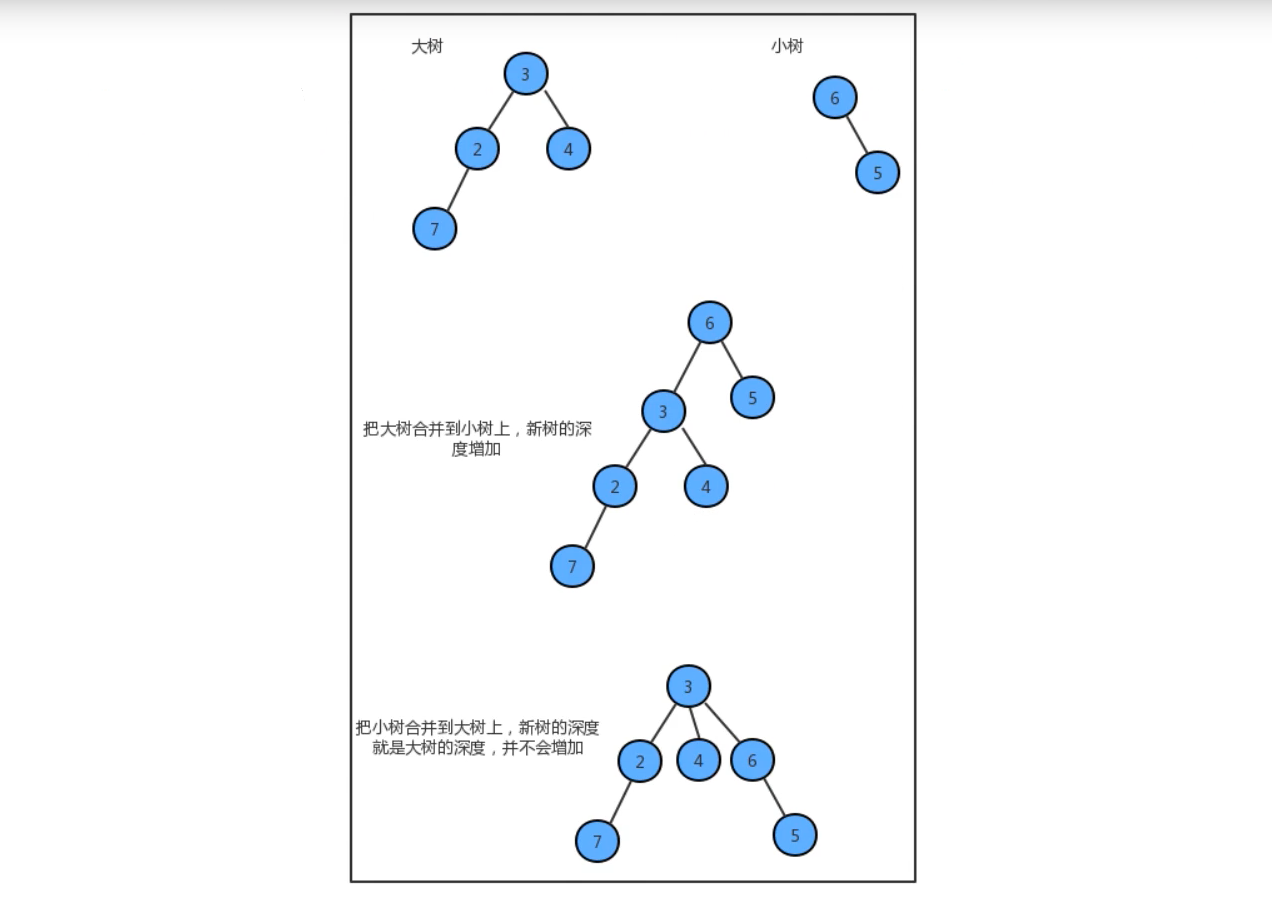### 并查集(Disjoint-Set)

xiaohai 2022-03-30 15:05:04 285人围观 标签： 算法

### 4.13.1、并查集的定义

• 查询元素p和元素q是否属于同一组
• 合并元素p和元素q所在的组### 4.13.2、并查集的结构

• 每个元素都唯一的对应一个结点
• 每一组数据中的多个元素都在同一棵树中
• 一个组中的数据对应的树和另一个组的数据对应的树之间没有任何联系
• 元素在树中并没有子父级关系的硬性要求### 4.13.3、并查集API设计

private int count：记录并查集中数据的分组个数

public boolean connected(int p,int q)：判断并查集中元素p和q是否在同一个分组中
public int find(int p)：元素p所在分组的标识符
public void union(int p,int q)：把p元素所在分组和q元素所在分组合并

### 4.13.4、并查集实现

``````package cn.test.algorithm.datastruct;

public class UF {
//元素和组
private int[] eleAndGroup;

//组的个数
private int count;

public UF(int N) {
//初始化分组的数量
count = N;

eleAndGroup = new int[N];

//初始化eleAndGroup的元素和所在分组的标识，让eleAndGroup数组的索引作为并查集的每个结点的元素，并且让每个索引处的值作为组标识
for (int i = 0; i < eleAndGroup.length; i++) {
eleAndGroup[i] = i;
}
}

//分组个数
public int count() {
return count;
}

//查找p元素的分组标识符
public int find(int p) {
return eleAndGroup[p];
}

//判断并查集元素p和q是否在一个分组中
public boolean connected(int p, int q) {
return find(p) == find(q);
}

//合并两个组的元素
public void union(int p, int q) {
if (connected(p, q)) {
return;
}

//找到p所在组的标识符
int pGroup = find(p);
//找到q所在组的标识符
int qGroup = find(q);
//合并组，让p所在组的所有元素的标识符变为q所在分组的标识符
for (int i = 0; i < eleAndGroup.length; i++) {
if (eleAndGroup[i] == pGroup) {
eleAndGroup[i] = qGroup;
}
}

//分组个数减1
count--;
}
}
``````

``````package cn.test.algorithm.test;

import cn.test.algorithm.datastruct.UF;

import java.util.Scanner;

public class UFTest {
public static void main(String[] args) {
//创建并查集对象
UF uf = new UF(100);

//从控制台录入两个合并的元素，调用union进行合并，获取合并后的并查集的分组数是否减少和组是否一样
Scanner scanner = new Scanner(System.in);
while (true) {
System.out.println("请输入第一个要合并的元素：");
int p = scanner.nextInt();
System.out.println("请输入第二个要合并的元素：");
int q = scanner.nextInt();

//判断两个是否在同一个组
if (uf.connected(p, q)) {
System.out.println("（" + p + "," + q + "）两个元素在同一个分组了");
continue;
} else {
uf.union(p, q);
System.out.println("当前并查集中还有：" + uf.count() + "个分组");
}
}

}
}
``````

``````请输入第一个要合并的元素：
1

3

1

3
（1,3）两个元素在同一个分组了

1

2

1

2
（1,2）两个元素在同一个分组了

``````

### 4.13.6、并查集算法优化

• 仍然让eleAndGroup数组的索引作为某个结点的元素
• eleAndGroup[i]的值不再是当前结点所在分组的标识，而是该结点的父结点``````package cn.test.algorithm.datastruct;

public class UF_Tree {
//元素和组
private int[] eleAndGroup;

//组的个数
private int count;

public UF_Tree(int N) {
//初始化分组的数量
count = N;

eleAndGroup = new int[N];

//初始化eleAndGroup的元素和所在分组的标识，让eleAndGroup数组的索引作为并查集的每个结点的元素，并且让每个索引处的值作为组标识
for (int i = 0; i < eleAndGroup.length; i++) {
eleAndGroup[i] = i;
}
}

//分组个数
public int count() {
return count;
}

//查找p元素的分组标识符
public int find(int p) {
while (true) {
if (p == eleAndGroup[p]) {
return p;
}
p = eleAndGroup[p];
}
}

//判断并查集元素p和q是否在一个分组中
public boolean connected(int p, int q) {
return find(p) == find(q);
}

//合并两个组的元素
public void union(int p, int q) {
//找到p所在组的标识符
int pRoot = find(p);
//找到q所在组的标识符
int qRoot = find(q);

//如果p和q在同一个分组就返回
if (pRoot == qRoot) {
return;
}

//让p所在树的根结点的父结点为q所在树的根节点即可
eleAndGroup[pRoot] = qRoot;

//分组个数减1
count--;
}
}
``````

``````package cn.test.algorithm.test;

import cn.test.algorithm.datastruct.UF_Tree;

import java.util.Scanner;

public class UFTreeTest {
public static void main(String[] args) {
//创建并查集对象
UF_Tree uf = new UF_Tree(100);

//从控制台录入两个合并的元素，调用union进行合并，获取合并后的并查集的分组数是否减少和组是否一样
Scanner scanner = new Scanner(System.in);
while (true) {
System.out.println("请输入第一个要合并的元素：");
int p = scanner.nextInt();
System.out.println("请输入第二个要合并的元素：");
int q = scanner.nextInt();

//判断两个是否在同一个组
if (uf.connected(p, q)) {
System.out.println("（" + p + "," + q + "）两个元素在同一个分组了");
continue;
} else {
uf.union(p, q);
System.out.println("当前并查集中还有：" + uf.count() + "个分组");
}
}

}
}
``````

``````请输入第一个要合并的元素：
1

3

1

2

1

2
（1,2）两个元素在同一个分组了

``````

### 4.13.7、路径压缩法``````//存储每个根结点对应的树中的元素个数
private int[] sz;
``````

``````package cn.test.algorithm.datastruct;

public class UF_Tree_Weighted {
//元素和组
private int[] eleAndGroup;

//存在每个根结点对应树中的保存结点的个数
private int[] sz;

//组的个数
private int count;

public UF_Tree_Weighted(int N) {
//初始化分组的数量
count = N;

eleAndGroup = new int[N];
sz = new int[N];

//初始化eleAndGroup的元素和所在分组的标识，让eleAndGroup数组的索引作为并查集的每个结点的元素，并且让每个索引处的值作为组标识
for (int i = 0; i < eleAndGroup.length; i++) {
eleAndGroup[i] = i;
sz[i] = 1;//默认情况下都是1
}
}

//分组个数
public int count() {
return count;
}

//查找p元素的分组标识符
public int find(int p) {
while (true) {
if (p == eleAndGroup[p]) {
return p;
}
p = eleAndGroup[p];
}
}

//判断并查集元素p和q是否在一个分组中
public boolean connected(int p, int q) {
return find(p) == find(q);
}

//合并两个组的元素
public void union(int p, int q) {
//找到p所在组的标识符
int pRoot = find(p);
//找到q所在组的标识符
int qRoot = find(q);

//如果p和q在同一个分组就返回
if (pRoot == qRoot) {
return;
}

//判断proot对应的大还是qroot对应的树大，最终需要把小的合并到大的上
if(sz[pRoot] < sz[qRoot]){
eleAndGroup[pRoot] = qRoot;
sz[qRoot] += sz[qRoot];
}else{
eleAndGroup[qRoot] = pRoot;
sz[pRoot] += sz[qRoot];
}

//分组个数减1
count--;
}
}
``````

``````package cn.test.algorithm.test;

import cn.test.algorithm.datastruct.UF_Tree_Weighted;

import java.util.Scanner;

public class UFTreeWeightedTest {
public static void main(String[] args) {
//创建并查集对象
UF_Tree_Weighted uf = new UF_Tree_Weighted(100);

//从控制台录入两个合并的元素，调用union进行合并，获取合并后的并查集的分组数是否减少和组是否一样
Scanner scanner = new Scanner(System.in);
while (true) {
System.out.println("请输入第一个要合并的元素：");
int p = scanner.nextInt();
System.out.println("请输入第二个要合并的元素：");
int q = scanner.nextInt();

//判断两个是否在同一个组
if (uf.connected(p, q)) {
System.out.println("（" + p + "," + q + "）两个元素在同一个分组了");
continue;
} else {
uf.union(p, q);
System.out.println("当前并查集中还有：" + uf.count() + "个分组");
}
}

}
}
``````

``````请输入第一个要合并的元素：
1

3

1

3
（1,3）两个元素在同一个分组了

1

2

1

4

``````